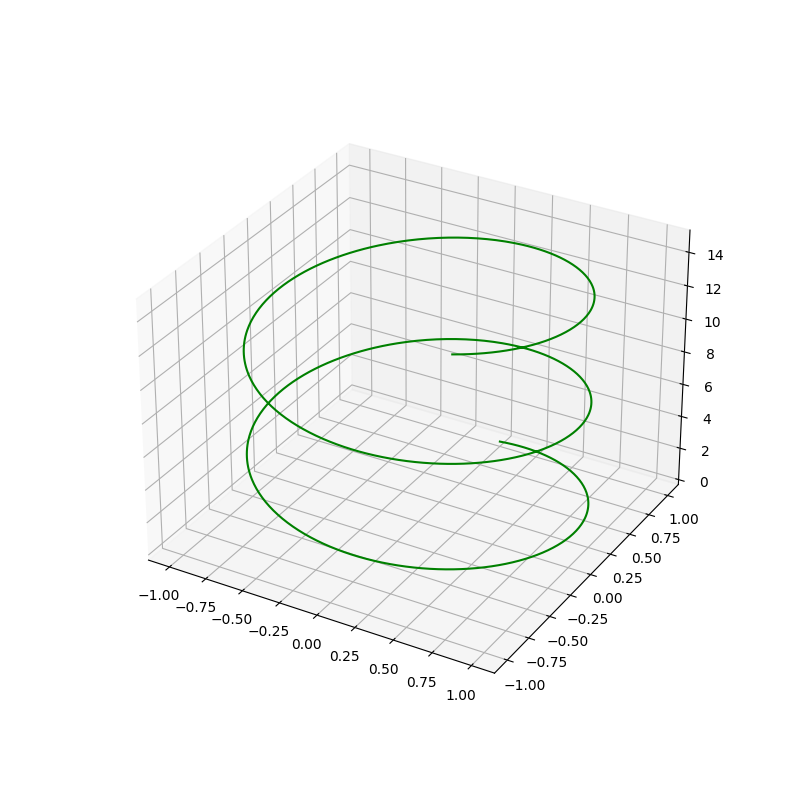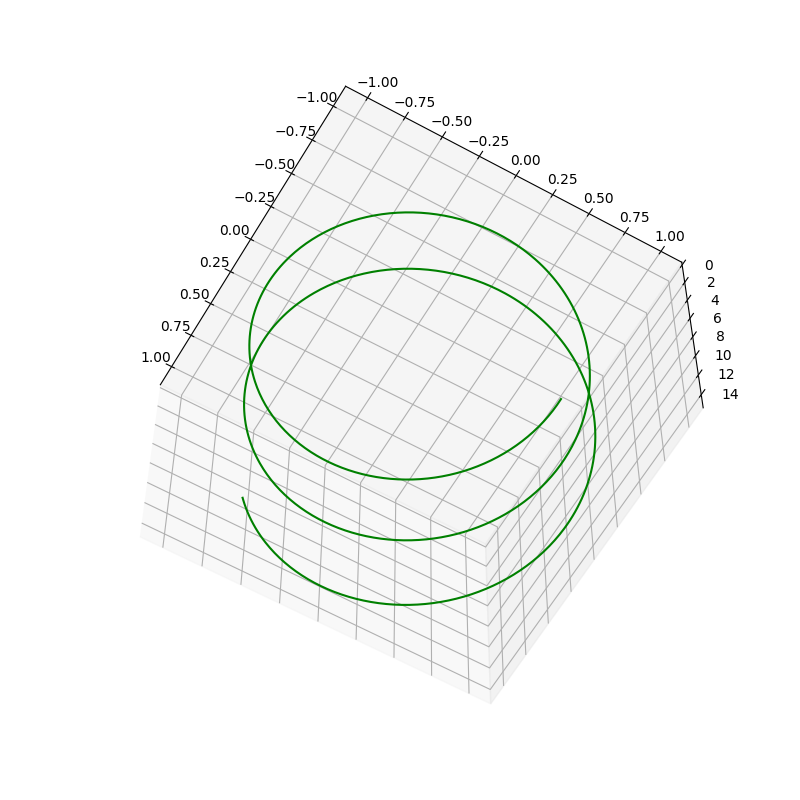import numpy as np
import matplotlib.pyplot as plt

fig = plt.figure(figsize = (8, 8))
ax = plt.axes(projection = '3d')

# Data for a three-dimensional line
z = np.linspace(0, 15, 1000)
x = np.sin(z)
y = np.cos(z)
ax.plot3D(x, y, z, 'green')
plt.show()
plt.show()## view_init() 视角转换

view_init(elev=None, azim=None, vertical_axis='z')

import numpy as np
import matplotlib.pyplot as plt

fig = plt.figure(figsize = (8, 8))
ax = plt.axes(projection = '3d')

# Data for a three-dimensional line
z = np.linspace(0, 15, 1000)
x = np.sin(z)
y = np.cos(z)
ax.plot3D(x, y, z, 'green')

#视角
#ax.view_init(120, 30, 'x')
#ax.view_init(120, 30, 'y')
ax.view_init(120, 30, 'z')

plt.show()Last modification：April 24th, 2022 at 01:48 pm